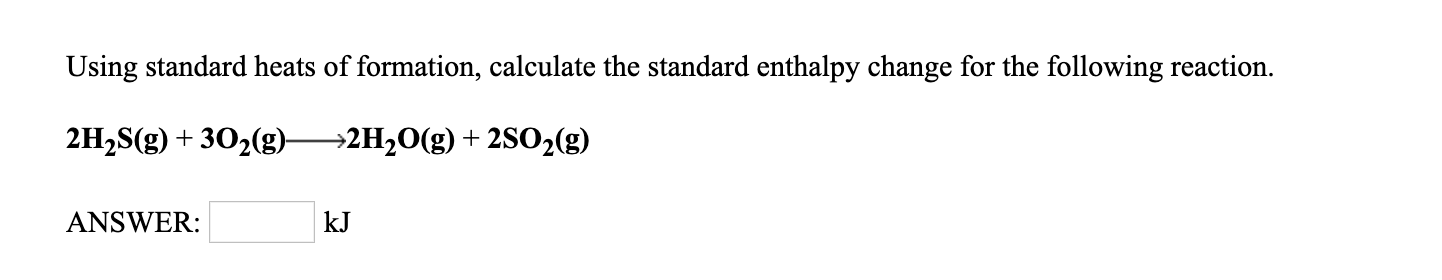Using standard heats of formation, calculate the standard enthalpy change for the following reaction.2H2S(g) +302(g)^2H20(g) + 2S02(g)ANSWER:kJ

Questionhelp_outlineImage TranscriptioncloseUsing standard heats of formation, calculate the standard enthalpy change for the following reaction. 2H2S(g) +302(g)^2H20(g) + 2S02(g) ANSWER: kJ fullscreen
Step 1

The exchange of heat between the system and the surrounding is equal to the change in enthalpy of the reaction. The change in enthalpy is the difference between the enthalpy of the product and the enthalpy of the reactant.

The heat change when 1 mole of a compound in the standard state is formed from its constituent element is known as standard enthalpy of formation (∆ H°f). The superscript degree represents the standard state conditions and the subscript f represents the formation.

The enthalpy of a reaction carried out at the standard condition is known as the standard enthalpy of the reaction.

Consider the general reaction represented in equation (1).

The standard enthalpy change for the reaction represented in equation (1) is calculated by using the expression shown in equation (2).

In general, equation (2) is represented as shown in equation (3), in which n and m represent the stoichiometric coefficients of the products and the reactants, respectively.

Step 2

The given reaction is represented by equation (4).

The standard heat of formation values for the compounds is shown in table (a).

Step 3

The standard enthalpy change for the given reaction is calculated by using the expression given in equation (5).

Substitute -241.8 for ∆H°f (H2O), -296.1 kJ/mol for ∆H°f (SO2), -20.1 for ∆H°f (H2S), and 0 kJ...

Want to see the full answer?

See Solution

Want to see this answer and more?

Our solutions are written by experts, many with advanced degrees, and available 24/7

See Solution
Tagged in

Physical Chemistry